×
###### 塞浦路斯證券交易委員會（CySEC）

Skilling Ltd獲得塞浦路斯證券交易委員會（CySEC）監管，CIF許可證號357/18

###### 塞席爾金融服務管理局（FSA）

Skilling (Seychelles) Ltd獲得塞席爾金融服務管理局（FSA）授權，並受其監管，許可證號SD042

# What are Bollinger Bands?

Bollinger Bands (BB) were developed by the technical analyst John Bollinger as a volatility indicator. They consist of two Bands, drawn below and above a simple moving average (SMA) of any market. Bollinger Bands were designed to show a trader when a large move might be expected. The bands are drawn around a SMA with a standard deviation, which is a mathematical formula that measures price volatility, giving a visual representation of how the price might vary from its current value.

Calculations for Bollinger Bands:

• an N-period moving average (MA)
• an upper band at K times an N-period standard deviation above the moving average (MA + Kσ)
• a lower band at K times an N-period standard deviation below the moving average (MA − Kσ)

Typical values for N and K are 20 and 2, respectively.

If the formula above seems complicated don’t worry too much; what is important is to understand the rationale behind it. As the two bands are dependent on the underlying instrument’s volatility, it follows that the bands automatically narrow when volatility is low, and widen when volatility increases. Please see the pictures below to better illustrate John Bollinger’s ideas.

### So, in more detail, according to Bollinger we can observe the following:

Diagram A
When the bands are contracting it indicates that we can expect major price movements.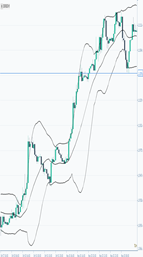Diagram B
When the bands are far apart and the price breaks through these, and then comes back within the bands, that should indicate that the current trend is ending.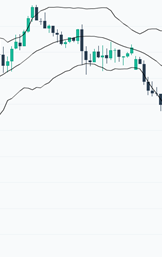Diagram C
If the price moves outside the bands, we can expect a continuation of the current trend.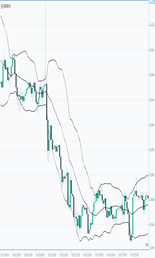Diagram D
If the price touches one of the bands, it follows that the price will reach the other band at some point too.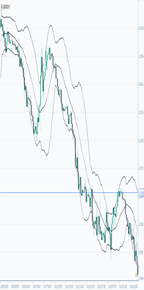### Interpretation of Bollinger Bands

As per the above we can see that Bollinger Bands are able to indicate major price movements and also give an indication as to whether prices are relatively too high or too low. However, they are not giving you a specific signal to buy or sell by themselves, hence, we suggest combining this with other indicators in order to help determine the expected direction of price movements and identify tops and bottoms. The use of Bollinger Bands becomes more practical when applied with RSI, MACD, Stochastic or Parabolic SAR

### Setting up Bollinger Bands in your Skilling platform

Period: The default set-up is 20, referring to the number of periods. You can change this as you see fit.

Deviations: Usually the standard deviation multiplier is set at two. Again, this can be tweaked to your preference.

Price inputs: The most commonly used price is the ‘Close’, but you can apply the ‘Open’, ‘Low’ and ‘High’ to determine the calculations.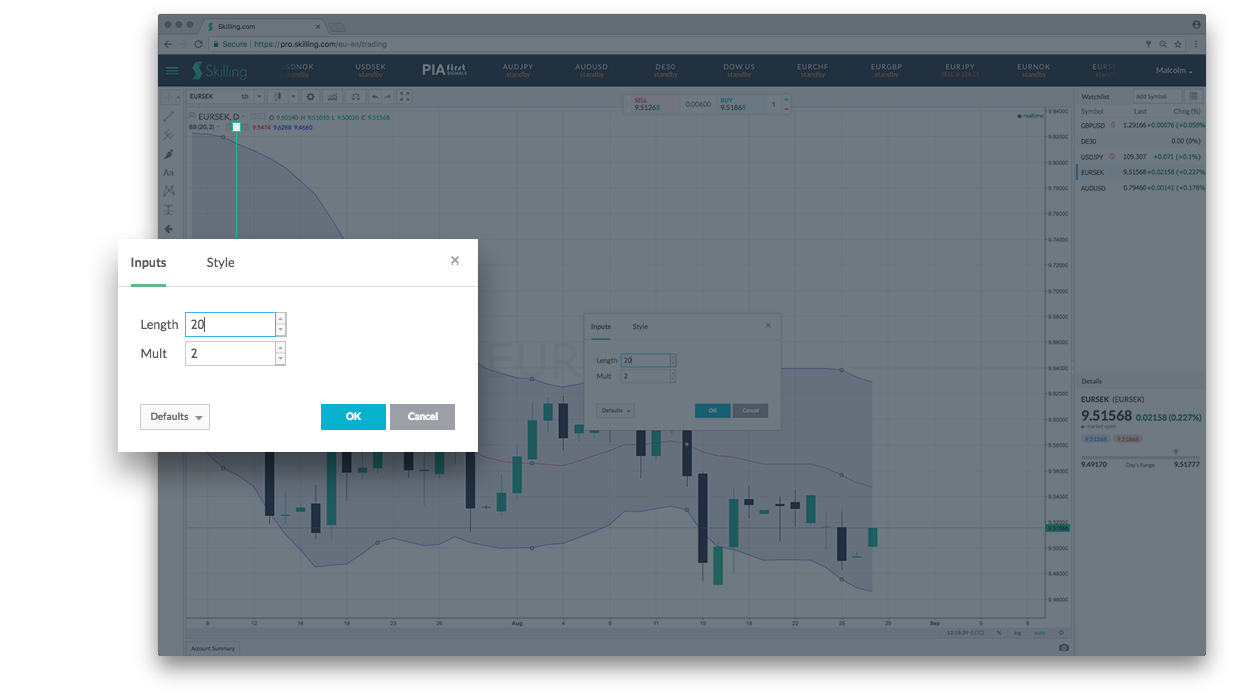### Skilling Summary

Bollinger Bands are very widely used by technical analysts and may be another indicator to make our top ten of important trader tools! Although the formula to work them out may appear daunting (which you anyway don’t need to learn to use them) the application of them is straightforward and logical. The premise is that markets go through periods of calm (low volatility) and excitement (high volatility). We think all traders should, at least be familiar, with Bollinger Bands!